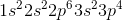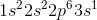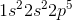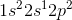Physical Chemistry : Electron Configuration

Example Questions

Example Question #1 : Electron Configuration

Which of the following electron configurations indicates an atom in an excited state?Explanation:

An atom is considered to be in an excited state when one of the electrons has jumped to a higher energy level while a lower energy level is available. In the case of, an electron has jumped to the 2p energy level while there is still room in the lower 2s subshell. As a result, it is considered to be in an excited state.

Example Question #36 : Nuclear, Quantum, And Molecular Chemistry

What is the complete ground state electron configuration for the magnesium atom?

1s22s22p63s2

1s22s22p23s2

1s22s23s2

1s42p63s2

1s22s22p63s6

1s22s22p63s2

Explanation:

Magnesium has an atomic number of 12, so the total number of electrons in its configuration should add up to twelve. The maximum number of electrons in the s subshell is two. Of all the answer choices, only 1s22s22p63s2 fits the criteria. The sum of the exponent values is 12, matching the atomic number of magnesium, and the number of electrons in the s and p subshells matches the maximum amount possible.

Example Question #37 : Nuclear, Quantum, And Molecular Chemistry

What is the complete ground state electron configuration for the iron atom?

1s22s22p63s23p64s23d4

1s22s22p63s23p63d6

1s22s22p63s24s23d6

1s42s22p63s23p24s23d6

1s22s22p63s23p64s23d6

1s22s22p63s23p64s23d6

Explanation:

Iron has an atomic number of 26, so the total number of electrons in its configuration should add up to twenty six. The maximum number of electrons in the s subshell is two. The sum of the exponent values is 26, matching the atomic number of magnesium, and the number of electrons in the s and p subshells matches the maximum amount possible.

Example Question #38 : Nuclear, Quantum, And Molecular Chemistry

In an atom or molecule, why can't two electrons have the same four electronic quantum numbers?

Kinetic energy operator

The first law of thermodynamics

The Pauli Exclusion Principle

Heisenberg Uncertainty Principle

Harmonic Reaction Orders